Christoffel-Schwarz formula

The formula(*)

constituting an integral representation for a function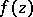which defines a conformal mapping of the upper half-plane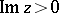onto the interior of a bounded polygon with vertices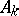and vertex angles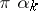(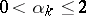,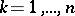). Moreover,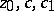are certain constants, and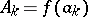. The constant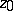can be fixed arbitrarily in the upper half-plane. A triple of points in the sequence, say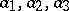, can be prescribed arbitrarily; the remaining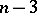pointsand the constantsare uniquely determined if the vertices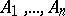of the polygon are prescribed (see ). Formula (*) was established independently by E.B. Christoffel (1867, see ) and H.A. Schwarz (1869, see ). The integral on the right-hand side of (*) is known as a Christoffel–Schwarz integral.

The basic difficulty in using formula (*) is to find the unknown parameters. No general methods are known for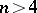.

Methods have been worked out for approximating the parameters of the Christoffel–Schwarz formula (see , ).

The Christoffel–Schwarz formula remains valid for polygons with one or more vertices at infinity. In that case the angle between the sides at infinity is, by definition, the angle (with minus sign) between the relevant sides (or their continuations) at a finite point. If the pre-image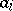of one of the vertices is the point at infinity, the corresponding factor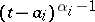is dropped in formula (*).

The Christoffel–Schwarz formula is also valid for a function that maps the unit disconto the above polygon. In that case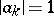,,. Modifications of the formula are valid for functions mapping the upper half-plane — or the interior and exterior of the unit disc — onto the exterior of a polygon (see ).

The Christoffel–Schwarz formula may be generalized to the case in whichdefines a conformal mapping of a circular annulus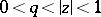, or, in general, a multiply-connected domain defined by deletingdiscs from the interior of a disc, onto a domain (multiply-connected of the same degree) bounded by polygons (see , ).

How to Cite This Entry:
Christoffel-Schwarz formula. Encyclopedia of Mathematics. URL: http://encyclopediaofmath.org/index.php?title=Christoffel-Schwarz_formula&oldid=46343
This article was adapted from an original article by Yu.D. Maksimov (originator), which appeared in Encyclopedia of Mathematics - ISBN 1402006098. See original article